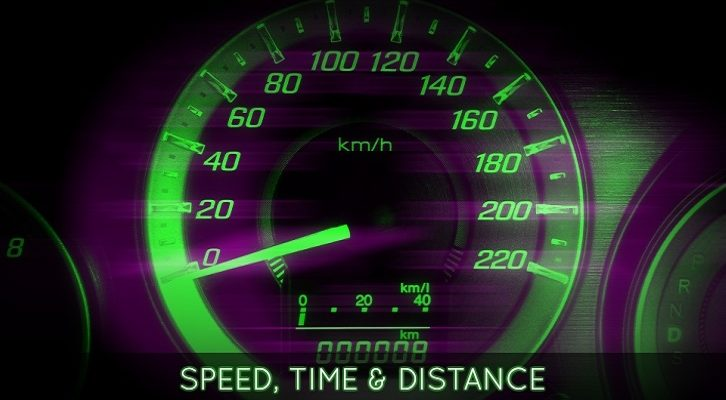# Races – Solutions

Have given below solutions to the races questions that can be found here.

1. Two friends A and B simultaneously start running around a circular track . They run in the same direction. A travels at 6m/s and B runs at b m/s. If they cross each other at exactly two points on the circular track and b is a natural number less than 30, how many values can b take?

Let track length be equal to T. Time taken to meet for the first time = T / relative speed
= T/(6-b) or T/(b-6)

Time taken for a lap for A = T/6
Time taken for a lap for A = T/b

So, time taken to meet for the first time at the starting point = LCM (T/6, T/b) = T / HCF (6,b)

Number of meeting points on the track = Time taken to meet at starting point/Time taken for first meeting = Relative speed / HCF (6,b). For a more detailed discussion on this have a look at the last few slides in this presentation.

So, in essence we have to find values for b such that 6-b/ HCF(6,b) = 2 or b-6/ HCF(6,b) = 2

b = 2, 10, 18 satisfy this equation. So, there are three different values that b can take.

2. Three friends A, B and C decide to run around a circular track. They start at the same time and run in the same direction. A is the quickest and when A finishes a lap, it is seen that C is as much behind B as B is behind A. When A completes 3 laps, C is the exact same position on the circular track as B was when A finished 1 lap. Find the ratio of the speeds of A,B and C?

Let track length be equal to T. When a completes a lap, let us assume B has run a distance of (t-d). At this time, C should have run a distance of (t-2d)

After three laps C would have traveled a distance of 3 * (t-2d) = 3t – 6d.

After 3 laps C is in the same position as B was at the end one lap. So, the position after 3t-6d should be the same as t-d. Or, C should be at a distance of d from the end of the lap. C will have completed less than 3 laps (as he is slower than A), so he could have traveled a distance of either t-d or 2t-d.

=> 3t-6d = t-d => 2t = 5d => d = 0.4t => The distances covered by A,B and C when A completes a lap will be t, 0.6t and 0.2t respectively. Or, the ratio of their speeds is 5:3:1

In the second scenario, 3t-6d = 2t-d => t = 5d=> d = 0.2t => The distances covered by A,B and C when A completes a lap will be t, 0.8t and 0.6t respectively. Or, the ratio of their speeds is 5:4:3

The ratio of the speeds of A, B and C is either 5:3:1 or 5:4:3